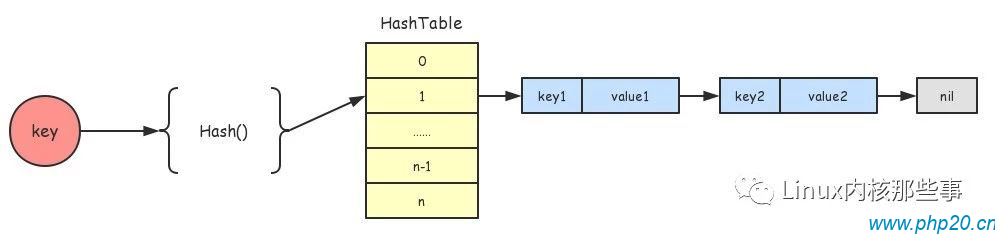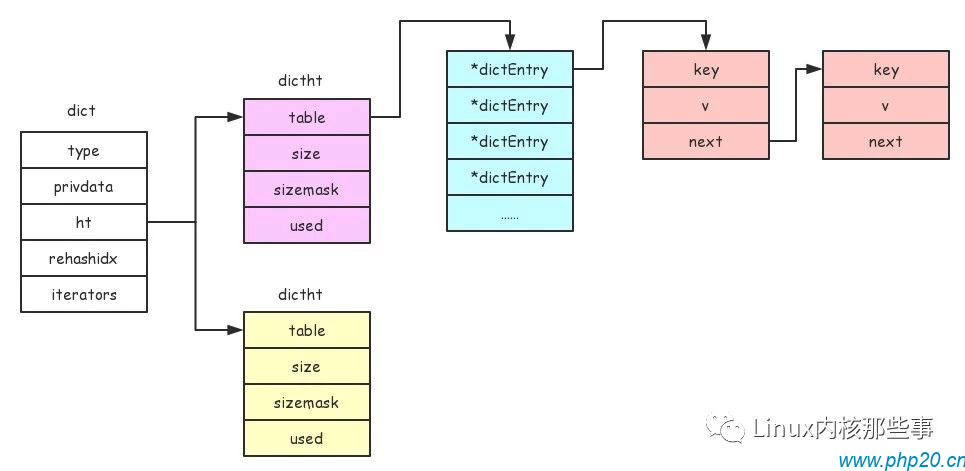# Redis字典设计详解

`Redis` 是一个高性能的 `key-value` 内存数据库，与 `Memcached` 只能存储字符串数据类型不一样，它支持存储的数据结构类型包括：字符串（string）、链表（lists）、哈希表（hash）、集合（set）、有序集合（zset）等。

`Redis` 的高性能得益于其 `I/O事件驱动` 模型，当然本文并不是讨论 `Redis` 的所有知识点，下面主要介绍 `Redis` 的核心数据结构：`字典` 的设计和实现。

### 哈希表介绍

`Redis` 本质上就是在字典上挂载着各种数据结构，我们先来看看 `字典` 这种数据结构。Redis的 `字典` 其实是就是 `哈希表（HashTable）`，我们来看看 `哈希表` 的定义：• 通过Hash函数把key映射到哈希表的某个位置

• 如果有不同的key被映射到同一个位置（冲突），采用拉链法（使用链表存储）来处理。

### Redis字典介绍

Redis的字典基本遵从了哈希表的设计，当然在通用性和性能上改进了一些地方，下面我们来解释一下Redis字典的设计。

```  dictEntry {
*key;
{
*val;
u64;
s64;
d;
} v;
dictEntry *next;
} dictEntry;

dictht {
dictEntry **table;
size;
used;
} dictht;

dict {
dictType *type;
*privdata;
dictht ht[];
rehashidx;
iterators;
} dict;```#### dict结构

• `type`：是用户自定义的函数列表，主要用于插入数据到字典时进行的一些操作，比如计算key哈希值的 `hashFunction` 函数句柄。

• `privdata`：创建字典时指定的私有数据，一般提供给 `type` 字段中的函数句柄使用。

• `ht`：类型为 `dictht` 结构的数组，这个数组有两个元素，而 `dictht` 结构主要用于保存数据，至于为什么需要两个 `dictht` 结构是因为rehash的需要。

• `rehashidx`：rehash操作过程中最后一次处理的桶索引。

• `iterators`：用于迭代字典中的数据。

#### dictht结构

• `table`：类型为 `dictEntry` 结构指针的数组，用于保存数据，每个 `key-value` 的数据对通过类型为 `dictEntry` 的结构来存储。

• `size`：table数组的大小。

• `sizemark`：用于取模，得到一个 `0 ～ size` 的索引值。

• `used`：表示字典中有多少个元素。

#### dictEntry结构

• `key`：数据的键，主要用于查找数据。

• `v`：数据的值，数据主要通过这个字段来存储。

• `next`：用于解决Hash冲突，把冲突的key连接起来（拉链法）。

### 字典的创建

```dict *(dictType *type,
*privDataPtr)
{
dict *d = ((*d));

(d,type,privDataPtr);
d;
}

(dict *d, dictType *type,
*privDataPtr)
{
(&d->[]);
(&d->[]);
d-> = type;
d-> = privDataPtr;
d-> = -;
d-> = ;
DICT_OK;
}

(dictht *ht)
{
ht-> = ;
ht-> = ;
ht-> = ;
ht-> = ;
}```

`dictCreate()` 函数申请了一个类型为 `dict` 的结构，然后通过调用 `_dictInit()` 函数对其进行初始化操作，总体过程比较简单。下面来看看在Redis中怎么创建一个字典的：

```dictType commandTableDictType = {
dictSdsCaseHash,
,
,
dictSdsKeyCaseCompare,
dictSdsDestructor,

};

() {
...
server. = (&commandTableDictType,);
...
}```

```  dictType {
(*hashFunction)(  *key);
*(*keyDup)( *privdata,   *key);
*(*valDup)( *privdata,   *obj);
(*keyCompare)( *privdata,   *key1,   *key2);
(*keyDestructor)( *privdata,  *key);
(*valDestructor)( *privdata,  *obj);
} dictType;```

`dictType` 结构的每个字段都是一个函数指针，下面说明一下各个字段的作用：

• `hashFunction`：用于计算键的哈希值

• `keyDup`：用于复制数据的键

• `valDup`：用于复制数据的值

• `keyCompare`：用于比较键是否相等

• `keyDestructor`：用于释放复制出来的键的内存

• `valDestructor`：用于释放复制出来的值的内存

### 插入数据到字典

``` (dict *d,  *key,  *val)
{
dictEntry *entry = (d,key,);

(!entry)  DICT_ERR;
(d, entry, val);
DICT_OK;
}```

`dictAdd()` 函数主要还是通过调用 `dictAddRaw()` 函数来完成插入操作，`dictAddRaw()` 函数的代码如下：

```dictEntry *(dict *d,  *key, dictEntry **existing)
{
;
dictEntry *entry;
dictht *ht;

((d)) (d);

(( = (d, key, (d,key), existing)) == -)
;

ht = (d) ? &d->[] : &d->[];
entry = ((*entry));
entry-> = ht->[];
ht->[] = entry;
ht->++;

(d, entry, key);
entry;
}```

`dictAddRaw()` 函数主要完成以下几个工作：

• 判断是否正在进行rehash操作（`dictIsRehashing()` 判断为真），如果是就调用 `_dictRehashStep()` 函数进行rehash。

• 通过调用 `_dictKeyIndex()` 函数计算key对应所在哈希表的位置（索引）`index`

• 如果正在rehash，那么就使用ht数组的第二个哈希表，否则就用第一个（原因下面会说明）。

• 创建一个 `dictEntry` 结构用于保存数据的键和值。

`dictAddRaw()` 函数会返回一个类型为 `dictEntry` 结构的值，然后 `dictAdd()` 函数通过调用 `dictSetVal()` 函数设置其值。

### Rehash操作Redis在插入数据到字典时，会通过 `_dictExpandIfNeeded()` 函数来判断是否需要进行Rehash操作，`_dictExpandIfNeeded()` 函数代码如下：

```  (dict *d)
{
((d))  DICT_OK;

(d->[]. == )  (d, DICT_HT_INITIAL_SIZE);

(d->[]. >= d->[]. &&
(dict_can_resize ||
d->[]./d->[]. > dict_force_resize_ratio))
{
(d, d->[].*);
}
DICT_OK;
}```

`_dictExpandIfNeeded()` 函数主要完成3个工作：

• 通过 `dictIsRehashing()` 来判断字典是否正在Rehash操作，如果是就直接返回OK，不需要再进行Rehash。

• 如果字典的第一个哈希表的大小为0，表示需要对第一个哈希表进行初始化。

• 如果第一个哈希表的元素个数大于等于哈希表的大小，那么就对第一个哈希表进行Rehash操作（把哈希表的大小扩大为原来的2倍）。

``` (dict *d,   size)
{
((d) || d->[]. > size)
DICT_ERR;

dictht n;
realsize = (size);

(realsize == d->[].)  DICT_ERR;

n. = realsize;
n. = realsize-;
n. = (realsize*(dictEntry*));
n. = ;

(d->[]. == ) {
d->[] = n;
DICT_OK;
}

d->[] = n;
d-> = ;
DICT_OK;
}```

`dictExpand()` 函数比较简单，就是申请一个更大的哈希数组，如果第一个哈希表的哈希数组为空，那么就把第一个哈希表的哈希数组设置为新的哈希数组。否则将第二个哈希表的哈希数组设置为新的哈希数组。

``` (dict *d,  n) {
empty_visits = n*;
(!(d))  ;

(n-- && d->[]. != ) {
dictEntry *de, *nextde;

(d->[]. > ( )d->);
(d->[].[d->] == ) {
d->++;
(--empty_visits == )  ;
}
de = d->[].[d->];
(de) {
h;

nextde = de->;
h = (d, de->) & d->[].;
de-> = d->[].[h];
d->[].[h] = de;
d->[].--;
d->[].++;
de = nextde;
}
d->[].[d->] = ;
d->++;
}

(d->[]. == ) {
(d->[].);
d->[] = d->[];
(&d->[]);
d-> = -;
;
}

;
}```

`dictRehash()` 函数的第二个参数是指定了每次要对多少个槽进行Rehash（也就是冲突链表），Rehash操作就是遍历第一个哈希表的所有数据，然后重新计算key的哈希值，保存到第二个哈希表中，并且从第一个哈希表中删除。当第一个哈希表的元素个数为0时，就将第一个哈希表替换成第二个哈希表，并且完成Rehash操作。

### 查找数据

```dictEntry *(dict *d,   *key)
{
dictEntry *he;
h, idx, table;

(d->[]. + d->[]. == )  ;
((d)) (d);
h = (d, key);
(table = ; table <= ; table++) {
idx = h & d->[table].;
he = d->[table].[idx];
(he) {
(key==he-> || (d, key, he->))
he;
he = he->;
}
(!(d))  ;
}
;
}```

• 如果字典为空（第一个和第二个哈希表都为空），那么就返回NULL。

• 如果正在进行Rehash操作，那么就调用 `_dictRehashStep()` 对数据进行分批Rehash。

• 计算key的哈希值，并且先在第一个哈希表中查找是否存在，如果存在就返回key对应的值。

• 如果key不在第一个哈希表中，那么就要判断当前是否正在Rehash操作，如果是就在第二哈希表中查找key是否存在。因为在Rehash的过程中，key有可能被移动到第二个哈希表中。

## mysql存储过程下分割字符串函数

2022-3-2 14:24:14

## mysql批量更新表前缀

2022-3-2 14:30:46